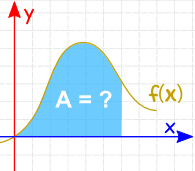# Integration Rules

## Integration

Integration can be used to find areas, volumes, central points and many useful things. But it is often used to find the area underneath the graph of a function like this:The integral of many functions are well known, and there are useful rules to work out the integral of more complicated functions, many of which are shown here.

Common Functions Function Integral
Constant a dx ax + C
Variable x dx x2/2 + C
Square x2 dx x3/3 + C
Reciprocal (1/x) dx ln|x| + C
Exponential ex dx ex + C
ax dx ax/ln(a) + C
ln(x) dx x ln(x) − x + C
Trigonometry (x in radians) cos(x) dx sin(x) + C
sin(x) dx -cos(x) + C
sec2(x) dx tan(x) + C

Rules Function
Integral
Multiplication by constant cf(x) dx cf(x) dx
Power Rule (n≠-1) xn dx xn+1n+1 + C
Sum Rule (f + g) dx f dx + g dx
Difference Rule (f - g) dx f dx - g dx
Integration by Parts See Integration by Parts
Substitution Rule See Integration by Substitution

## Examples

### Example: what is the integral of sin(x) ?

From the table above it is listed as being −cos(x) + C

It is written as:

sin(x) dx = −cos(x) + C

### Example: what is the integral of 1/x ?

From the table above it is listed as being ln|x| + C

It is written as:

(1/x) dx = ln|x| + C

The vertical bars || either side of x mean absolute value, because we don't want to give negative values to the natural logarithm function ln.

### Example: What is ∫x3 dx ?

The question is asking "what is the integral of x3 ?"

We can use the Power Rule, where n=3:

xn dx = xn+1n+1 + C

x3 dx = x44 + C

### Example: What is ∫√x dx ?

√x is also x0.5

We can use the Power Rule, where n=½:

xn dx = xn+1n+1 + C

x0.5 dx = x1.51.5 + C

### Example: What is ∫6x2 dx ?

We can move the 6 outside the integral:

6x2 dx = 6x2 dx

And now use the Power Rule on x2:

= 6 x33 + C

Simplify:

= 2x3 + C

### Example: What is ∫cos x + x dx ?

Use the Sum Rule:

cos x + x dx = cos x dx + x dx

Work out the integral of each (using table above):

= sin x + x2/2 + C

### Example: What is ∫ew − 3 dw ?

Use the Difference Rule:

ew − 3 dw =ew dw − 3 dw

Then work out the integral of each (using table above):

= ew − 3w + C

### Example: What is ∫8z + 4z3 − 6z2 dz ?

Use the Sum and Difference Rule:

8z + 4z3 − 6z2 dz =8z dz + 4z3 dz − 6z2 dz

Constant Multiplication:

= 8z dz + 4z3 dz − 6z2 dz

Power Rule:

= 8z2/2 + 4z4/4 − 6z3/3 + C

Simplify:

= 4z2 + z4 − 2z3 + C